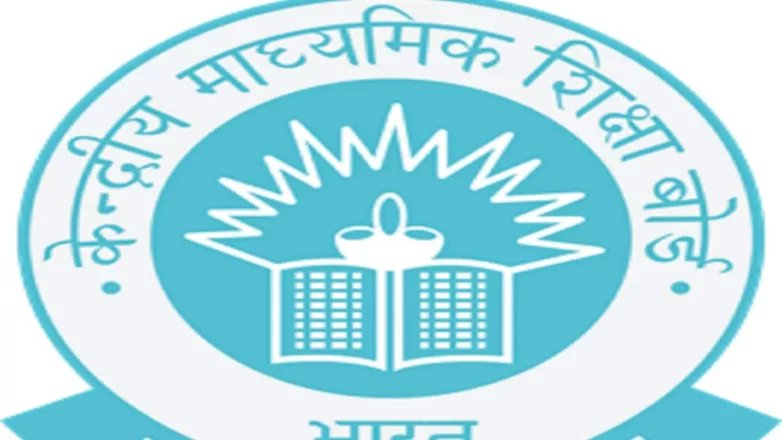By | 17 Sep 2022 at 9:56 AMCBSE Sample Paper 2023: The Central Board of Secondary Education (CBSE) has released the sample question paper of class 10th and 12th 2022-23. This time only one test will be taken. In addition to practical exam, there will be theory exam. According to the board, there will be questions in five sections in class X. The first section will be of objective type questions. This time 20 questions of one mark each will be asked. The examination of science subject in class X will be of 70 marks. There will be 39 questions in it. At the same time, there will be an examination of 80 marks for social science. 37 questions will be asked in it.

12th sample paper

The sample paper of class 12 has also been released. In class 12th, 35-35 questions will be asked in Physics and Chemistry. There will be 18 objective type questions. Seven questions of two marks each will be asked. 70 marks questions will be asked in Biology. There will be 33 questions in it. The number of objective type questions will be 16 and the number of two marks questions will be five. In class 12, 40 questions will be asked in Mathematics. The 80 marks exam will consist of 18 questions of one mark and five questions of two marks. It is to be known that due to corona infection in 2022, the board exam was taken in two terms. In this, the examination of 50 marks for each subject was taken in the first term and the examination of 50 marks was taken in the second term. But the board has decided to take the 2023 exam on the old pattern.

The exam will start from 15th February 2023. Prior to this, practical examination will be taken in January.

Rajeev Ranjan (City Coordinator CBSE) said, “The board has released both the sample paper and the marking scheme. This time the exam will be held from February 15. Preparation is being done at the school level. The sample paper will facilitate the students in preparation.

Number of questions in 10th will be more than 12th

This time the number of questions in 10th will be more than 12th. 39 questions will be asked in Science and 37 questions will be asked in Social Science. According to the board, 37 questions will be asked in Mathematics and 30 questions in English. This time questions will be asked from the entire syllabus. So the board has released the sample paper so that the students can prepare from now. Along with the sample paper, the board has also released the marking scheme. Students will also be able to understand the method of answering in it.